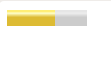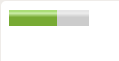# How to specify the optimal value for the gauge in HTML5?

• Last Updated : 08 Mar, 2021

The task is to specify an optimal value for the gauge. The optimal value is used to indicate the range of meters of a meter. The value must be within the range i.e between min and max. It is used to define the measurement of scale in a well-defined range with a frictional value. It is also known as a gauge.

Approach:

• Add a meter in body using meter tag.
• Set the optimum value in meter tag.

Syntax

`<meter optimum = "value"></meter>  `

Note: It contains the floating point number which represent the optimal value of the gauge.

Example 1:

## HTML

 `<``html``>``   ``<``body``> ``      ``<``meter` `value``=``"0.6"``         ``max``=``"1"``         ``min``=``"0"``         ``optimum``=``"0.5"``         ``high``=``"0.5"``         ``low``=``"0.2"``> ``      `` ``   `````

OutputExample 2: Same as above just change the optimum value

## HTML

 ` ``<``html``>``   ``<``body``> ``      ``<``meter` `value``=``"0.6"``         ``max``=``"1"``         ``min``=``"0"``         ``optimum``=``"0.6"``         ``high``=``"0.5"``         ``low``=``"0.2"``> ``      `` ``   `````

OutputMy Personal Notes arrow_drop_up